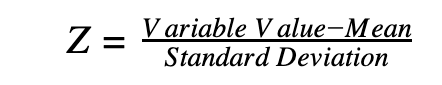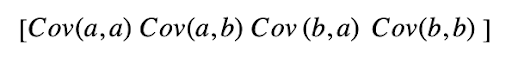Need a discount on popular programming courses? Find them here. View offers

Don't have an account?

Have you read our submission guidelines?

Go back to

# Principal Component Analysis

With this ever-changing world of technology, it's essential to stay up-to-date with the advancements in the field of technology. Machine Learning and Artificial Intelligence is an ongoing trend in the market nowadays. Principal Component Analysis is also one of them; this blog on Principal Component Analysis helps you understand the science behind handling high dimensional data efficiently.

In the real-world on the day to day basis, we need to analyze complex data, i.e., multi-dimensional data. Plotted data and various hidden patterns are studied and analyzed to train some machine learning tools.

## What is Principal Component Analysis?

Principal Component Analysis (PCA) is a dimensionality reduction technique that enables you to identify correlations and patterns in a data set so that it can be transformed into a data set of significantly lower dimensions without loss of any important information.

The main motive to implement PCA is to figure out unique patterns and correlations in the given data set. When a strong correlation is found between data sets and variables, a final decision is made about reducing the data so that the final data can be constructed with the significant data still retained.

PCA is a process that is essential in solving data-driven problems which are highly complex and which involves the use of high-dimensional data sets.

### The Necessity of Principal Component Analysis

Machine learning works best when the data given to the trainer or the machine is large and concise. Having a large amount of data is usually good for better results and accuracy since we have large data to train the machine with, but it comes with its own set of problems and pitfalls. The worst being the curse of dimensionality.

In high dimensional sets of data, there can be many inconsistencies in the features and redundant data in the dataset, which increases the time of computation of the machine.

To get rid of these problems, we needed a process to simplify the data and still to retain significant data for machine learning. Dimensionality reduction techniques can be used to filter only a limited number of significant features needed for training, and this is where PCA comes in.

### Computation of Principal Component Analysis

The below steps are followed to perform dimensionality reduction using PCA:

1. Standardization of the data
2. Computing the covariance matrix
3. Calculating the eigenvectors and eigenvalues
4. Computing the Principal Components
5. Reducing the dimensions of the data set

Let us discuss each of the steps in detail:

#### 1. Data Standardization

In Data Analysis and Processing, standardization is really important, without standardization of data, results we get will most probably be biased and inaccurate. Standardization is scaling data in a way that all the variables and their values lie within a similar range.

For example, let us take two datasets, one between 25-200 and the other has a value between 100-2000. In such a case, the result we will get from these predictor values will be biased due to uneven intervals, since the dataset with higher range will have more impact on the outcome of the machine.

Therefore, standardization into a comparable range is really important. Standardization is carried out by subtracting each value in the data from the mean and dividing it by the overall deviation in the data set.#### 2. Covariance Matrix Computation

PCA helps to identify the correlation and dependencies among the features in a data set. Correlation between the different variables in the data set is expressed through a Covariance Matrix. It is important to identify heavily dependent variables which h contain redundancy and biased information, which can alter with the outcome and reduce the performance of any specific system.

In mathematics, a covariance matrix is a P ×P matrix, where p represents the dimensions of any specified dataset.

Let us consider a case where we have a 2-D data set with "a" and "b" being the two variables; then the covariance matrix will be denoted by

##We can draw some conclusions from the above matrix:

• Cov(a, a) is the covariance of the variable with itself, which is the variance of a.
• Cov(a,b) shows us the covariance of “a” with respect to “b”. Since covariance is commutative, Cov(a,b) = Cov(b,a)

#### 3. Eigenvectors and Eigenvalues Calculation

Eigenvectors and eigenvalues are the mathematical constructs that must be computed from the covariance matrix to determine the principal components of the data set.

But what are principal components of a data set, let's understand more about principal components first,

What are Principal Components

Principal Components can be described as the new set of variables that are obtained from the processing of the initial ser of variables. Principal components are computed, such s the new set of variables that are highly significant and independent of each other. These new sets of variables possess the most important and useful information that was scattered in the initial stage amongst the initial variables.

If you have a data set which is of 5 dimensions, then 5 principal components are computed from those dimensions, such that the first principal component stores the maximum possible information, and the second one stores the remaining maximum info, and so on, you get the idea.

Now coming back to Eigenvectors and Eigenvalues,

Eigenvectors and Eigenvalues are the two algebraic formulations that are always computed in the pair. For every eigenvector, there is an eigenvalue. The dimensions in the data determine the number of eigenvectors that you need to calculate.

Let us consider a 2-D data set for which the two eigenvectors are computed with their eigenvalues. The idea behind the computation for the eigenvectors and eigenvalues is to know where in the data, there lies the most variance. As the more the variance, the more information about that data, which possesses the way to enhance the system. Eigenvectors are used to identify and compute principal components.

Eigenvalues can also be explained as the scalars of the respective eigenvector. Therefore, eigenvectors and eigenvalues will compute the Principal Components of the data set.

#### 4. Principal Component Computation

Once finished with computation of our eigenvectors and eigenvalues, we have to arrange or order them in the decreasing order, where the eigenvector, which holds the most value, is the most significant and hence forms the first principal component of the system. Principal components of the lesser significance can be eliminated to reduce the dimensions of the data.

In the final step, we have to form a matrix called "feature matrix." It is an important step in the computation of the principal components. The feature matrix contains all the data variables that contain or possess maximum information about the data.

#### 5. Reducing the Dimensions of the Data Set

The last step in Principal Component Analysis is to rearrange the data with the final set of principal components, which represent the maximum and most significant information about the data set. You need to multiply the transpose of the original data set by the transpose of the obtained feature vector to replace the original data axis with the newly formed set of principal components.

That ends the theoretical process behind the PCA.

### Conclusion

PCA is a widely used and adaptive descriptive data analysis tool in its standard form. It also has many adaptations making it useful to a variety of data types and situations in several disciplines. Adaptations of PCA have been suggested for binary data, ordinal data, compositional data, discrete data, symbolic data, or data with special structure. PCA-related approaches play an important direct role in other statistical methods, such as linear regression and clustering of both individuals and variables. Methods such as correspondence analysis, canonical correlation analysis, or linear discriminant analysis might be loosely connected to PCA.

PCA has a vast literature and spans many disciplines. New adaptations and methodological results, as well as applications, are still appearing.

STAY IN LOOP TO BE AT THE TOP

#### Welcome to the club and Thank you for subscribing!

By Simran Kaur Arora

Simran works at Hackr as a technical writer. The graduate in MS Computer Science from the well known CS hub, aka Silicon Valley, is also an editor of the website. She enjoys writing about any tech topic, including programming, algorithms, cloud, data science, and AI. Traveling, sketching, and gardening are the hobbies that interest her.

View all post by the author

Disclosure: Hackr.io is supported by its audience. When you purchase through links on our site, we may earn an affiliate commission.